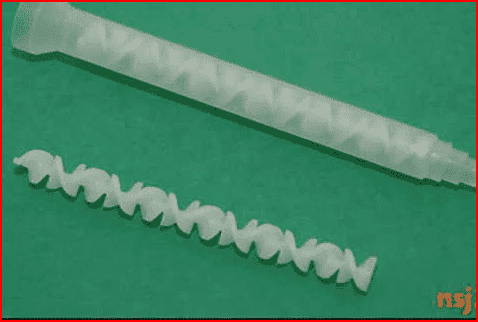# Looking for basic theory on mixing of gases

random_soldier
Hi,

In my situation, I am dealing with two ideal gases traveling down a pipe at the same bulk velocities. I am only considering a 1-D treatment at the moment and I would like to find out at which point the two gases should be considered a well mixed, homogeneous mixture. Would anyone know either the particular formulation or even the subject matter to consult to determine the well-mixing of gases?

Thanks and regards.

## Answers and Replies

Mentor
If they are just traveling down a straight pipe, the mixing will be slow. If that is okay for your application and you just want to quantify the (long) distance needed, I'll let others address that.

Instead, would it help your application to on-purpose encourage the mixing of the gasses to happen as soon as possible (as short a run of pipe as possible)? If so, you may be able to leverage some of the concepts for mixing liquids (like 2-part epoxies). See this previous PF thread for more information on that:https://i.ytimg.com/vi/JYW6QDldODk/hqdefault.jpg

random_soldier
It's to figure out how long I want the pipe segment to be in my CFD simulation so that I don't make a long segment adding unnecessary spatial meshing.

The real world setup the simulation is based on does not have provisions for applying the methods in that thread so no unfortunately. Thanks for the response though.

•berkeman
Gold Member
So you have a pipe and at the start of the pipe, the two gases are separated and you want to compute how much time/length it takes for them to mix perfectly?
You could solve the 1D convection-diffusion equation for the transport of species in time. In laminar flows, this can be done analytically. You could even consider the solution of the heat equation
$$C_t=C_{xx}$$
since there is an equivalence between transport of mass and heat. You then solve e.g. on the domain [-1,1] with boundary condition
$$C(x,0)=0, x<0$$
and:
$$C(x,0)=1, x>=0$$
For large t, you expect the concentration to go to ##C(x,\infty)=0.5##. You then compute the time it takes for the concentration to fulfill the condition ##C(x=1,t)=0.501##.

The mixing problem becomes more complicated when the species have large differences in fluid properties or if the problem is turbulent.

As a first guess, you could consider the concentration distribution in an infinitely large medium in time, where the initial mass is concentrated at x=0. The concentration in time is given by

$$C(x,t)=\frac{e^{-x^2/4Dt}}{\sqrt{4 \pi Dt}}$$
##D## is the binary diffusion coefficient of the two species. You can determine how much time it takes for the concentration at x=0 to reduce to 0.01. With the average pipe velocity, you can then determine the pipe length. For confined spaces, the mixing time increases. For instance in the semi-infinite domain ##x>0##, the concentration decay is twice as slow.

For turbulent flow problems, the mixing is much faster.

Last edited:
•random_soldier
Mentor
See Transport Phenomena by Bird, Stewart, and Lightfoot: Chapters on Mass Transport

••random_soldier, Lord Jestocost, bigfooted and 1 other person